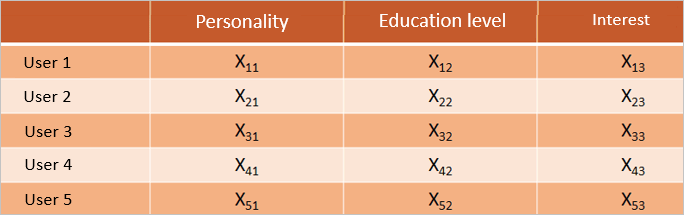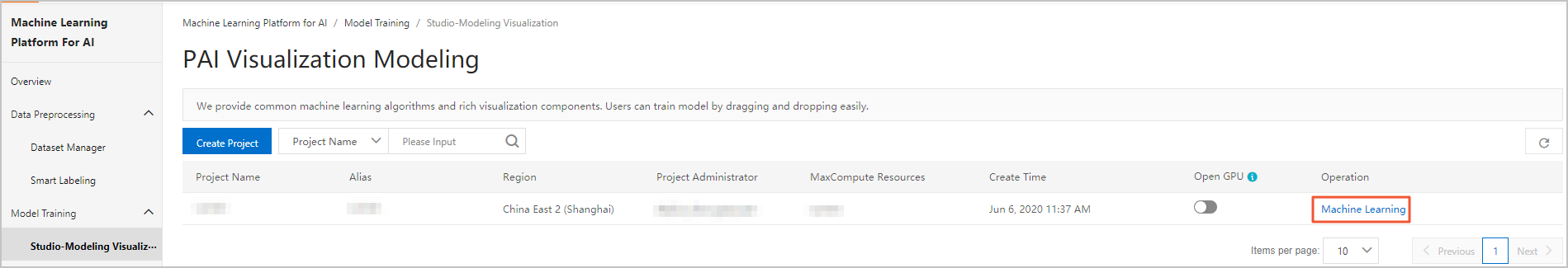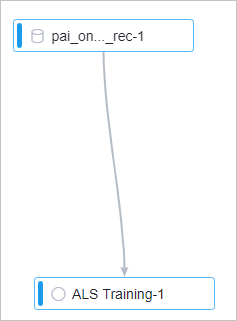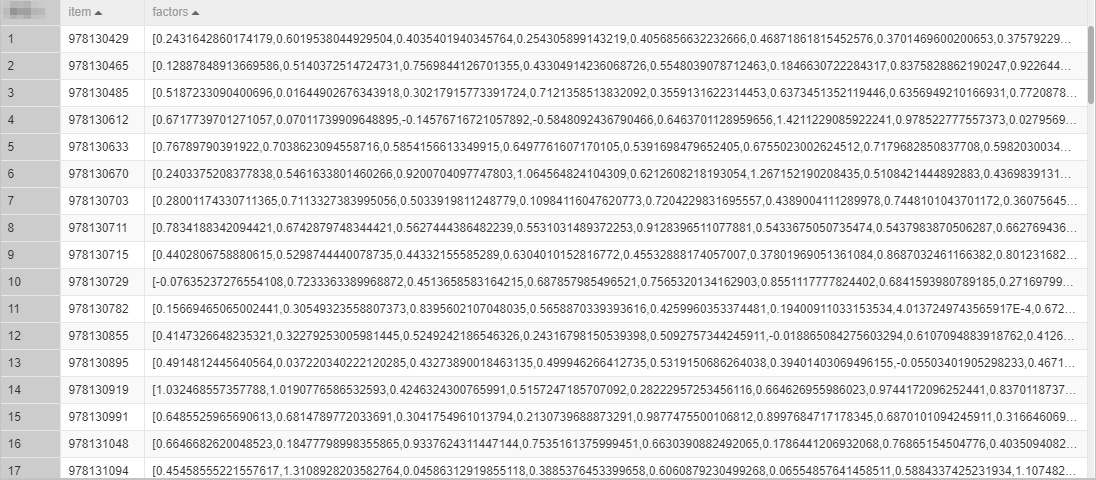Everyone has a rating system in their mind. The rating that a user assigns to a content item, such as a movie or a song, reflects whether the user likes or dislikes the content item. If a content provider can predict the ratings to be assigned by users to content, the provider can better understand user requirements. This way, the provider can recommend content to users more precisely. This topic describes how to use Alternating Least Squares (ALS), a factorization algorithm, to predict the ratings to be assigned by users to songs.

## Introduction to ALS

ALS factorizes models by using sparse matrix factorization and predicts the values of missing entries. This way, a basic model is trained. As a type of user-item collaborative filtering algorithm, ALS is also known as a hybrid collaborative filtering algorithm.

In this topic, ratings of songs are used as an example to introduce how ALS works. The source dataset, that is, Matrix A, contains the ratings that every user assigns to songs. The ratings may be sparse because not every user has listened to all the songs in the library and not all the songs in the library are rated by every user. The following figure shows Matrix A.ALS factorizes Matrix A to the product of the transposes of Matrix X and Matrix Y.
``Matrix A = Transpose of Matrix X × Transpose of Matrix Y``
The columns in Matrix X and rows in Matrix Y are known as factors in ALS. Assume that Matrix X and Matrix Y contain three factors: personality, education level, and interest. The following figure shows Matrix X that is factorized from Matrix A. Matrix X contains Vector M of each user.The following figure shows Matrix Y that is factorized from Matrix A. Matrix Y contains Vector N of each song.Based on the factorized data, ratings can be predicted. For example, to predict the rating to be assigned by User 6 to Song 3, you can multiply Vector M of User 6 in Matrix X by Vector N of Song 3 in Matrix Y.

## Procedure

1. Go to the Machine Learning Studio console.
1. Log on to the PAI console.
2. In the left-side navigation pane, choose Model Training > Studio-Modeling Visualization.
3. On the PAI Visualization Modeling page, find the project in which you want to create an experiment and click Machine Learning in the Operation column.2. Create and run an experiment.
1. In the left-side navigation pane, click Home.
2. In the Templates section, click Create below ALS music recommendations.
3. In the New Experiment dialog box, set the experiment parameters. You can use the default values of the parameters.
Parameter Description
Name The name of the experiment. Default value: ALS music recommendations.
Project The project in which you want to create the experiment. You cannot change the value of this parameter.
Description The description of the experiment. Default value: Using ALS to implement music and movie related content recommendations.
Save To The directory for storing the experiment. Default value: My Experiments.
4. Click OK.
5. Wait about 10 seconds. Then, click Experiments in the left-side navigation pane.
6. Click ALS music recommendations_XX under My Experiments. The canvas of the experiment appears.
My Experiments is the directory for storing the experiment that you created and ALS music recommendations_XX is the name of the experiment. In the experiment name, _XX is the ID that the system automatically creates for the experiment.7. In the top toolbar of the canvas, click Run.
The system automatically configures the data source of the experiment and parameters of the ALS component. Therefore, you can directly run the experiment. You can click each component on the canvas and view the component parameters in the right-side pane.
3. View the result of the experiment.
1. After the experiment is run, right-click ALS Training-1 on the canvas and choose View Data > View Output Port 1. In the dialog box that appears, view the data of Matrix X.2. Right-click ALS Training-1 on the canvas and choose View Data > View Output Port 2. In the dialog box that appears, view the data of Matrix Y.4. Predict ratings.
To predict the rating to be assigned by User 1 to Item 978130429, multiply Vector M of User 1 in Matrix X by Vector N of Item 978130429 in Matrix Y.
``````# Vector M
[0.5775652527809143,0.6737191677093506,0.9059759974479675,0.9866708517074585,0.15602371096611023,0.2735472023487091,0.4610620439052582,0.5312653183937073,0.3408969044685364,1.4993919134140015]
# Vector N
[0.2431642860174179,0.6019538044929504,0.4035401940345764,0.254305899143219,0.4056856632232666,0.46871861815452576,0.3701469600200653,0.3757922947406769,0.26486095786094666,0.37488409876823425]``````